Question

# NH4NO3 (s) NH4+ (aq) + NO3- (aq) H°f NH4NO3 (s) = -365.6 kJ/mol H°f NH4NO3 (aq)...

NH4NO3 (s)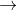NH4+ (aq) + NO3- (aq)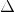H°f NH4NO3 (s) = -365.6 kJ/molH°f NH4NO3 (aq) = -339.9 kJ/mol

What isH°rxn?

We were unable to transcribe this image
We were unable to transcribe this image
We were unable to transcribe this image
We were unable to transcribe this image

using the given data to determine the ∆H°rxn.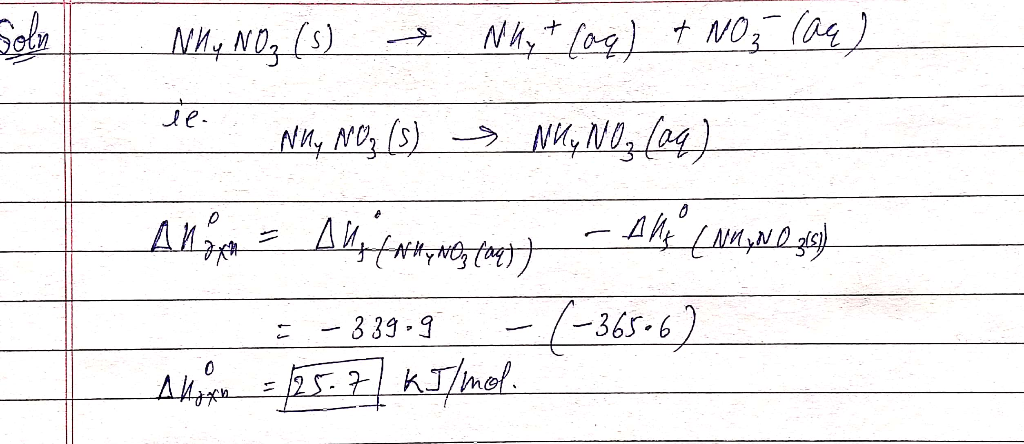#### Earn Coins

Coins can be redeemed for fabulous gifts.

Similar Homework Help Questions
• ### Given the information below, what is AGº for the reaction: NH4NO3(s) - NH4+ (aq) + NO3(aq)...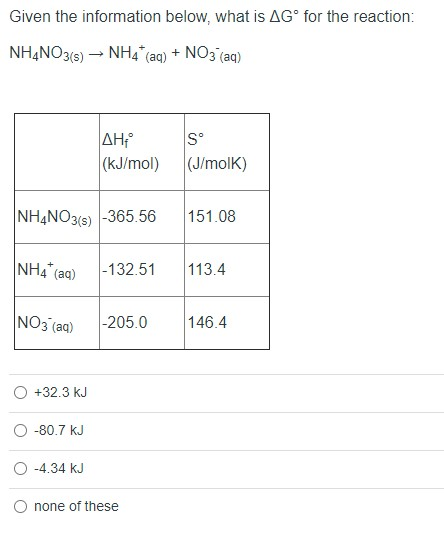Given the information below, what is AGº for the reaction: NH4NO3(s) - NH4+ (aq) + NO3(aq) AH (kJ/mol) S° (J/molk) NH4NO3(s) -365.56 151.08 NH4 (aq) -132.51 113.4 NO3(aq) -205.0 146.4 +32.3 kJ -80.7 kJ -4.34 kJ O none of these

• ### Determine the standard entropy change of the universe when 0.3 mol of NH4NO3 is produced, NH4...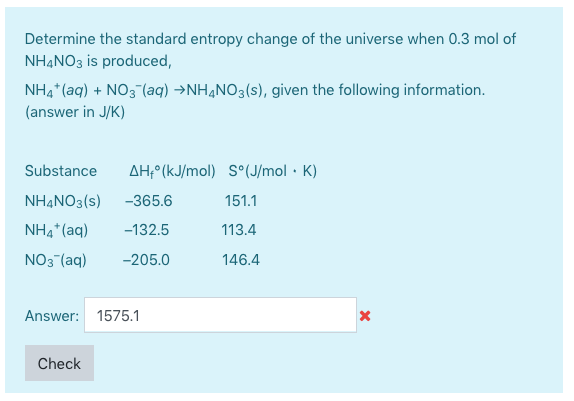Determine the standard entropy change of the universe when 0.3 mol of NH4NO3 is produced, NH4 (aq) NO3(aq) NH4NO3(s), given the following information. (answer in J/K) AH (kJ/mol) S°(J/mol K) Substance NH4NO 3(s) -365.6 151.1 NH4 (aq) -132.5 113.4 NO 3 (aq) -205.0 146.4 Answer: 1575.1 Check

• ### 1.) NH4NO3(s) ↔ NH4+(aq) + NO3-(aq) +17 kJ/mol what is K at room temp? A)0.993 B)1.00...

1.) NH4NO3(s) ↔ NH4+(aq) + NO3-(aq) +17 kJ/mol what is K at room temp? A)0.993 B)1.00 C)1.05 x 10-3 D)954 2.) For the following reactions: 2NH3(g) 3H2(g) + N2(g)   What is under the following conditions: T = 25oC PNH3 = 12.9 atm PH2 = 0.250 atm PN2 = 0.870 atm A) 33 kJ/mol B) 56 kJ/mol C) 10 kJ/mol D) 31 kJ/mol

• ### Determine the standard entropy change of the universe when 0.2 mol of NH,NO3 is produced, NH4+...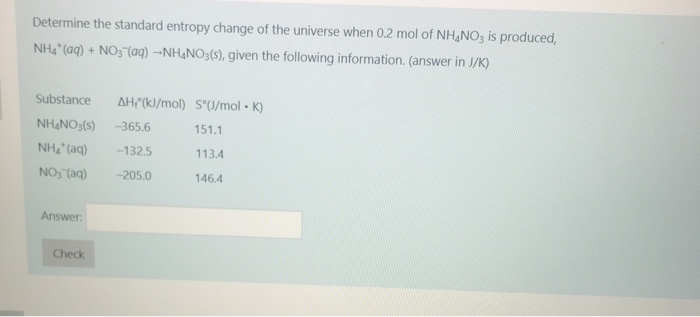Determine the standard entropy change of the universe when 0.2 mol of NH,NO3 is produced, NH4+ (aq) + NO3-(aq) --NH.NO3(s), given the following information. (answer in J/K) Substance NH.NO3(s) AH!"(kJ/mol) SºU/molK) -365.6 151.1 -132.5 113.4 -205.0 146.4 NH" (G) NO3(aq) Answer: Check

• ### Determine S°rxn for Zn (s) + 2HCl (aq) ZnCl2 (aq) + H2 (g).       Substance         ...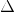Determine S°rxn for Zn (s) + 2HCl (aq) ZnCl2 (aq) + H2 (g).       Substance          S° (J/mol ⋅ K)       Zn(s)                     60.9       HCl(aq)                     56.5       H2(g)                     130.58       Zn2+(aq)                 −106.5       Cl−(aq)                     55.10 We were unable to transcribe this imageWe were unable to transcribe this image

• ### *** ** -305.01 -183.9 151.1 NH4NO3 (aq) -339.9 -190.6 259.8 AHºf (kJ/mol) AG°f (kJ/mol) Sº (/mol...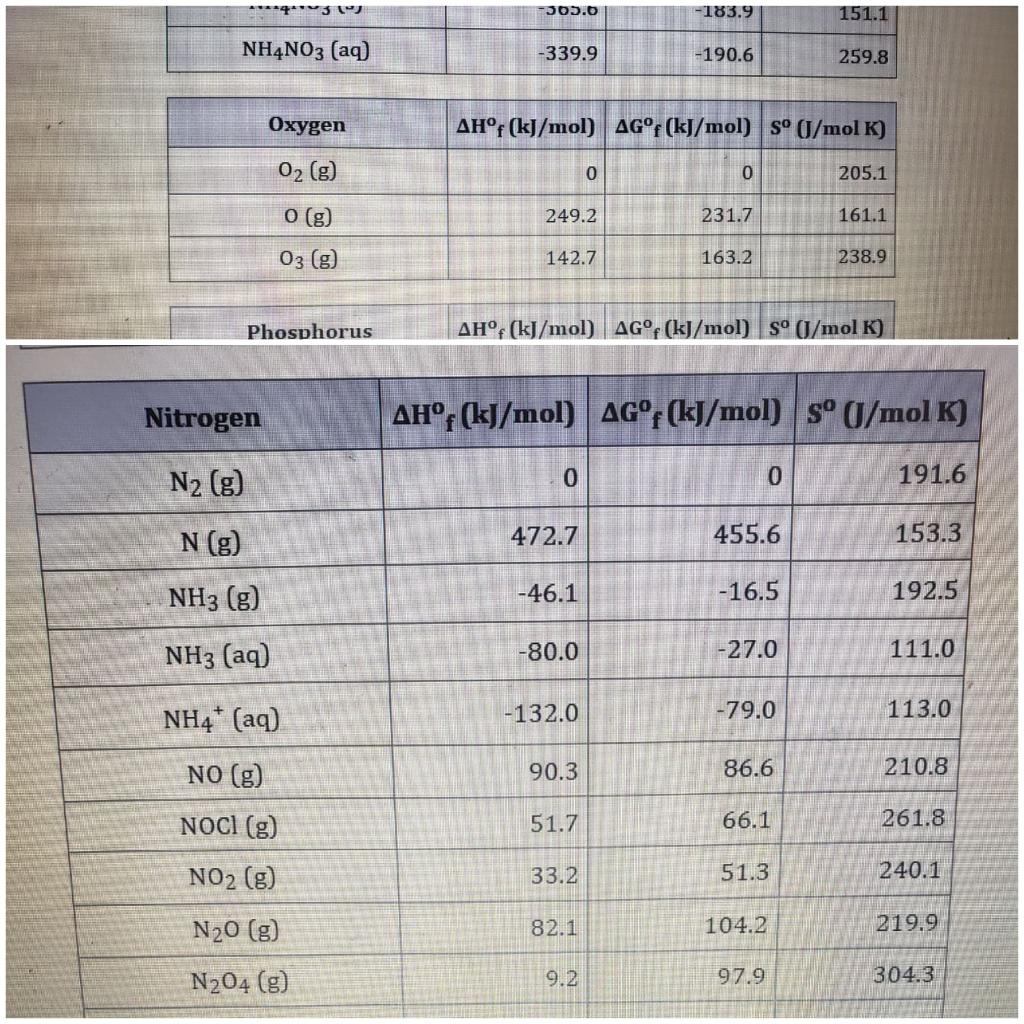*** ** -305.01 -183.9 151.1 NH4NO3 (aq) -339.9 -190.6 259.8 AHºf (kJ/mol) AG°f (kJ/mol) Sº (/mol K) 0 205.1 Oxygen 02 (g) O(g) 03 (g) 249.2 231.7 161.1 142.7 163.2 238.9 Phosphorus AH° (kJ/mol) AG°f(kJ/mol) sº (/mol K) Nitrogen AH°(kJ/mol) AG°f(kJ/mol) s° /mol K) N2 (8) 0 191.6 N (g) 472.7 455.6 153.3 NH3 (g) -46.1 -16.5 192.5 NH3 (aq) -80.0 -27.0 111.0 132.0 -79.0 113.0 90.3 86.6 210.8 51.7 66.1 261.8 NH4+ (aq) NO (g) NOCI (9) NO2 (g)...

• ### Nitrogen Ahºf (kJ/mol) AG°f (kJ/mol) Sº (J/mol K) 191.6 N2(9) 0 0 N(g) 472.7 455.6 153.3 NH3(g) -46.1 -16.5 192.5 NH3(a...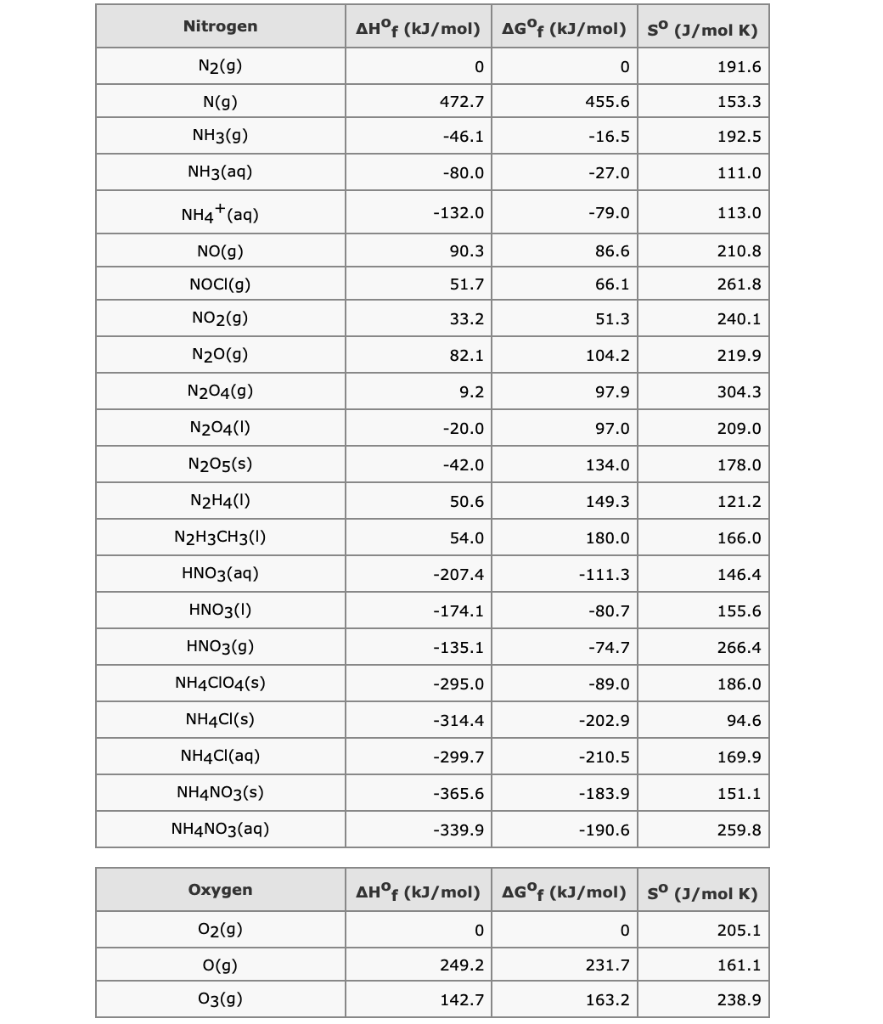Nitrogen Ahºf (kJ/mol) AG°f (kJ/mol) Sº (J/mol K) 191.6 N2(9) 0 0 N(g) 472.7 455.6 153.3 NH3(g) -46.1 -16.5 192.5 NH3(aq) -80.0 -27.0 111.0 NH4+ (aq) -132.0 -79.0 113.0 90.3 86.6 210.8 NO(9) NOCI(g) 51.7 66.1 261.8 NO2(g) 33.2 51.3 240.1 N20(9) 82.1 104.2 219.9 N204(9) 9.2 97.9 304.3 N204(0) -20.0 97.0 209.0 N205(s) -42.0 134.0 178.0 N2H4(0) 50.6 149.3 121.2 N2H3CH3 () 54.0 180.0 166.0 HNO3(aq) -207.4 -111.3 146.4 HNO3(1) -174.1 -80.7 155.6 HNO3(9) -135.1 -74.7 266.4 NH4ClO4(s) -295.0...

• ### HEM1043PO2-2010-12683 Determine the standard entropy change of the universe when 0.3 mol of NH4NO3 is produced,...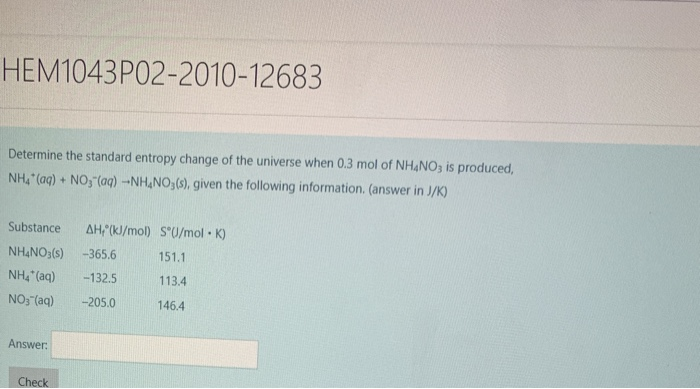HEM1043PO2-2010-12683 Determine the standard entropy change of the universe when 0.3 mol of NH4NO3 is produced, NH (aq) +NO,"(aq) -NH NO3(s), given the following information. (answer in J/K) AH (KI/mol) SU/mol K) Substance NH&NO3(s) -365.6 151.1 NH (aq) -132.5 113.4 NO3 (aq) -205.0 146.4 Answer Check

• ### When a 5.12-g sample of solid sodium nitrate dissolves in 31.1 g of water in a...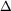When a 5.12-g sample of solid sodium nitrate dissolves in 31.1 g of water in a coffee-cup calorimeter (see above figure) the temperature falls from 25.00 oC to 16.37 oC. Calculate H in kJ/mol NaNO3 for the solution process. NaNO3(s) Na+(aq) + NO3-(aq) The specific heat of water is 4.18 J/g-K. We were unable to transcribe this imageWe were unable to transcribe this imagethermometer stirrer coffee cups containing reaction mixture When a 5.12-g sample of solid sodium nitrate dissolves in...

• ### Given: 1) NaOH Na+(aq) + OH- (aq) 2) NaOH + H+ Cl-(aq) H2O(l) + Na+(aq) +...

Given: 1) NaOH Na+(aq) + OH- (aq) 2) NaOH + H+ Cl-(aq) H2O(l) + Na+(aq) + Cl-(aq) 3) Na+(aq) + OH-(aq) + H+(aq) + Cl-(aq) H2O(l) + Na+(aq) + Cl-(aq) Show that equations 1 and 3 can be added algebraically to give equation 2. We were unable to transcribe this imageWe were unable to transcribe this imageWe were unable to transcribe this image